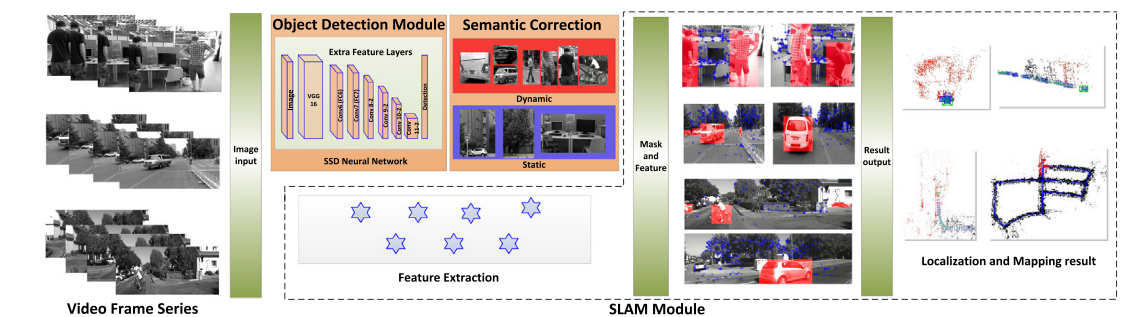[toc] # 2020 Dynamic-SLAM: Semantic monocular visual localization and mapping based on deep learning in dynamic environment **contributions**: • In view of the low recall rate of the existing SSD object detection network, a missed detection compensation algo- rithm based on the speed invariance in adjacent frames is proposed for SLAM system, which greatly improves the recall rate for detection and provides a good basis for the following module. • A selection tracking algorithm is proposed to eliminate the dynamic objects in a simple and effective way, which im- proves the robustness and accuracy of the system. • A feature-based visual Dynamic-SLAM system is constructed. Based on the SSD convolutional neural net- work, deep learning technology is constructed to a newly object detection thread, which combines prior knowledge to realize the detection of dynamic objects at semantic level in robot localization and mapping. （引用 https://zhuanlan.zhihu.com/p/128472528 这位大牛的翻译，翻译的挺好的, 如有侵权，请联系我删除：） 本文的主要三大贡献： 1. 针对SLAM系统提出了一种基于相邻帧速度不变性的丢失检测补偿算法，提高SSD的recall rate，为后续模块提供了良好的依据。 2. 提出了一种选择跟踪算法，以一种简单有效的方式消除动态对象，提高了系统的鲁棒性和准确性。 3. 构建了基于特征的可视化动态SLAM系统。 构建了基于SSD的目标检测模块线程，并将其检测结果作为先验知识提升SLAM性能。![Framework](https://cdn.jsdelivr.net/gh/yubaoliu/[email protected]/Dynamic-slam-freamework.png) **Missed detection compensation algorithm**(漏检补偿算法): 由于目标物体检测并不是那么精确的，会出现漏检等情况，此时可以通过前几帧的检测结果来对当前帧的目标物体的bounding box的位置进行预测。 论文中假设：动态物体在较短时间内的速度变化为常值，加速度为0. 论文中给出了计算公式，不过现在不清楚 $\Delta v$, $\Delta c_i(u,v)$是如何计算的, 以及最后那一项怎么得到？ ${}^Ka_i(u,v) = {}^{K-1}c_i(u,v)+\frac1k \sum_{f=K-1}^{K-k} \Delta^fc_i(u, v)\pm \frac12 a_{max}(u, v)$ ${}^K\hat c_i,(u,v) = {}^{K-1} c_i(u,v) + \frac1k \sum_{f=K-1}^{K-k} \Delta^fc_i(u,v)$ K代表当前帧，K-1为上一帧。 预测区域：${}^KA_i ({}^K a_{i,u}, {}^Ka_{i,v}, {}^{k-1} \hat w, {}^{K-1} \hat h)$ 中点坐标及长与宽 如果与预测区域不重叠，漏检的时候，则使用先前帧进行修补(添加到当前帧中)。 ${}^K \hat c_i (u,v) = {}^{K-1} c_i (u,v) + \frac1k \sum_{f=K-1}^{K-k} \Delta^f c_i(u,v)$ 问题：那么这个速度是怎么计算得到的呢？在文中我没有找到答案。 ![Missed detection compensation](https://cdn.jsdelivr.net/gh/yubaoliu/[email protected]/Dynamic-slam_missed_detection.png) 算法大概(Algorithm 1)： 遍历上一帧的所有bounding bos, 预测它们在下帧出现的问题。 在当前帧中遍历所有检测到的bouding box，即当前观测值。如果是在预测框范围内，则说明检测到了，反之则说明漏检了，用预测框修补上。 ![ALgorithm 1](https://cdn.jsdelivr.net/gh/yubaoliu/[email protected]/Dynamic-SLAM_Algorithm1.png) ![Dynamic characteristic score of common objects based on life experience](https://cdn.jsdelivr.net/gh/yubaoliu/[email protected]/Dynamic-SLAM_characteristic_score.png) **Seletive Tracking Method**（选择跟踪算法）： 本质就是去除动态点，在跟踪时只使用静态特征点。 calculating the aver- age pixel displacement $S_L(u, v)$ of the static feature points in the pixel region L. 使用静态的特征点来算出一个位移均值出来，这个值将作为差别是否为动态特征点的threshold. $\bar S_L(u,v) = \frac1{N_L} \sum_{i\in L} | \frac 1 Z_{s_i}K exp(\xi_k{}\hat{})P_{si} - \frac 1 Z_{s_i}K exp(\xi_{k-1}{}\hat{})P_{si} |$ 问题：哪些点是用作计算的静态特征点？应该先做个假设吧。从算法2 来看，可能是用的mask 为 0 的那些静态点，对应算法2 中的第4行。 （我的理解可能不对） ![Graphical representation of the selective tracking algorithm](https://cdn.jsdelivr.net/gh/yubaoliu/[email protected]/Dynamic-SLAM_selectiv_tracking_algorithm.png) 从算法2 中可看出，如果当前帧中bounding box中心点的位移变化范围大于上面那个threshold时，就可以判断此点为动态点。 ![ALgorithm2](https://cdn.jsdelivr.net/gh/yubaoliu/[email protected]/Dynamic-SLAM_algorithm2.png) # Conclusion This frame work has three major contributions. Firstly, in view of the low recall rate of the existing SSD object detection network, a missed detection compensation algorithm based on the speed invari- ance in adjacent frames is proposed for SLAM system, which greatly improves the recall rate for detection and provides a good basis for the following module. Secondly, a selective tracking algorithm is proposed to eliminate the dynamic objects in a simple and effective way, which improves the robustness and accuracy of the system. Finally, A feature-based visual Dynamic- SLAM system is constructed. Based on the SSD convolutional neural network, deep learning technology is constructed to a newly object detection thread, which combines prior knowledge to realize the detection of dynamic objects at semantic level in robot localization and mapping. # References - Xiao, L., Wang, J., Qiu, X., Rong, Z., & Zou, X. (2019). Dynamic-SLAM: Semantic monocular visual localization and mapping based on deep learning in dynamic environment. Robotics and Autonomous Systems, 117(April), 1–16. https://doi.org/10.1016/j.robot.2019.03.012 - [Paper阅读：Dynamic-SLAM](https://zhuanlan.zhihu.com/p/128472528)